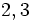# Element structure of special linear group:SL(2,5)

View element structure of particular groups | View other specific information about special linear group:SL(2,5)

This article gives detailed information about the element structure of special linear group:SL(2,5), which is a group of order 120.

## Conjugacy class structure

### Conjugacy classes

Nature of conjugacy class Eigenvalue pairs of all conjugacy classes Characteristic polynomials of all conjugacy classes Minimal polynomials of all conjugacy classes Size of conjugacy class Number of such conjugacy classes Total number of elements Semisimple? Diagonalizable over$\mathbb{F}_q$? Splits in$SL_2$ relative to$GL_2$? Representative matrices (one per conjugacy class)
Scalar$\{ 1, 1 \}$ or$\{ -1,-1\}$$x^2 - 2x + 1$ or$x^2 + 2x + 1$$x - 1$ or$x + 1$ 1 2 2 Yes Yes No$\begin{pmatrix} 1 & 0 \\ 0 & 1 \\\end{pmatrix}$ and$\begin{pmatrix} -1 & 0 \\ 0 & -1\\\end{pmatrix}$
Not diagonal, Jordan block of size two$\{ 1, 1 \}$ or$\{ -1,-1\}$$x^2 - 2x + 1$ or$x^2 + 3x + 1$$x^2 - 2x + 1$ or$x^2 + 2x + 1$ 12 4 48 No No Yes$\begin{pmatrix} 1 & 1 \\ 0 & 1 \\\end{pmatrix}$,$\begin{pmatrix} 1 & 2 \\ 0 & 1 \\\end{pmatrix}$,$\begin{pmatrix} -1 & 1 \\ 0 & -1 \\\end{pmatrix}$,$\begin{pmatrix} -1 & 2 \\ 0 & -1 \\\end{pmatrix}$
Diagonalizable over field:F25, not over field:F5. Must necessarily have no repeated eigenvalues. pair of square roots of$2$ in field:F25, pair of square roots of$3$ in field:F25$x^2 - x + 1$,$x^2 + x + 1$$x^2 - x + 1$,$x^2 + x +1$ 20 2 40 Yes No No$\begin{pmatrix}0 & -1\\ 1 & 1\\\end{pmatrix}$,$\begin{pmatrix}0 & -1\\ 1 & -1\\\end{pmatrix}$
Diagonalizable over field:F5 with distinct diagonal entries$2,3$$x^2 + 1$$x^2 + 1$ 30 1 30 Yes Yes No$\begin{pmatrix} 2 & 0 \\ 0 & 3 \\\end{pmatrix}$